# Quantificational LogicExamples

For convenience, we reproduce the item of Principia Metaphysica in which the Quantificational Logic is defined:In what follows, we give examples of the axioms and rules, consider some facts, and then draw out some consequences.

## Examples of the Axioms and Rules

Instances of Axiom 1:The first example asserts if everything exemplifies the property P, then if there is such a thing as a, then a exemplifies P. The second example says something similar. In formal terms, these examples tell us that we may instantiate the name a or variable z into a universal quantifier if we know that a or z denotes something. The third example asserts if a exemplifies every property, then if there is such a property as P, then a exemplifies P. The fourth example says something similar. In formal terms, examples 3 and 4 tell us that we may instantiate names of, and variables for, properties into universal claims if we know that the property name or variable has a denotation. The fifth example asserts if everything exemplifies P, then if there is something that is the x that exemplifies Q, then the x that exemplifies Q exemplifies P. The sixth example asserts If a exemplifies every property, then if there is such a property as being a y that bears R to the x that exemplifies Q, then a exemplifies the property of being a y that bears R to the x that exemplifies Q. In general, we may instantiate any term into a universal claim if we know that the term denotes something.

Note that in all the examples, the term instantiated into the universal claim is substitutable for the variable. This is designed to avoid the situation in which a term gets `captured', when substituted into a quantified claim, by a quantifier for that variable that already exists in the claim. For example, suppose the following claim is true: for every x, there is a y such that y is not identical with x. The variable y already appears in this claim bound by a quantifier. Now suppose we could instantiate the quantifier `for every x' in this claim to the variable y (variables are terms, so they can be instantiated into universal claims) by removing the quantifier from the claim and replacing y for x everywhere x appears. The resulting claim would be: there is a y such that y is not identical with y, which is clearly false. Therefore, we would have proven a falsehood from a truth. The problem with substituting the variable y in this claim is that it was `captured' by the quantifier `there is a y' that was already part of the claim. This alters the significance of the resulting sentence upon substitution, and the restriction that terms be substitutable for the quantified variable avoids this situation.

Instances of Axiom 2: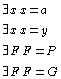The first example asserts there is something which is a. The second example asserts something similar. In general, these examples guarantee that every proper name such as a or variable such as y has a denotation. Similarly, the third example asserts there is such a property as P and the fourth asserts there is such a property as G. All primitive n-place constants and variables are governed by similar instances of Axiom 2. The last example asserts there is such a property as: being a y such that it is not the case that y exemplifies both P and Q. Any complex predicate free of definite descriptions will be governed by a similar instance of Axiom 2. Notice that the following are not instances of this axiom:Though given a particular interpretation, these sentences may in fact be true, but it is not a truth of logic that there is such a thing as the x that exemplifies Q; whether or not there is depends on the facts. Similarly, it is not a truth of logic that there is such a property as being a y that bears R to the x that exemplifies Q; whether or not there is again depends on whether there is something that uniquely exemplifies Q.

Instances of Axiom 3:The first example asserts If the x that exemplifies Q exemplifies P, then there is such a thing as the x that exemplifies Q. In formal terms, this tells us that if a definite description appears in a true atomic formula, then the description has a denotation. The second example asserts If a exemplifies the property of being a y such that y bears R to the x that exemplifies Q, then there is such a thing as the x that exemplifies Q. In formal terms, this tells us that even if the definite description appears embedded in a complex predicate, it must have a denotation for an atomic sentence involving the complex predicate to be true. Finally, the third example asserts if a exemplifies the property of being a y such that y bears R to the x that exemplifies Q, then there is such a thing as the property of being a y such that y bears R to the x that exemplifies Q. In formal terms, this tells us that the complex predicate itself must have a denotation if it appears in a true atomic formula, even if it has a definite description as a component.

Instances of Axiom 4: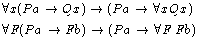The first example asserts if every x is such that if a exemplifies P then x exemplifies Q, then if Pa, then every x exemplifies Q . In formal terms, we can move a `vacuous' universal quantifier across a conditional. The restriction that the antecedent of the conditional contain no free occurences of the quantified variable prevents us from deriving a falsehood from a truth. For example, it is logically true that: every x is such that if x exemplifies P then x exemplifies P. But if we could move the quantifier across this conditional, we could conclude: if x exemplifies P, then every x exemplifies P. This is not a logical truth. It is false in those domains in which not all the elements are in the extension of P (in such domains, the sentence would be false when something that exemplifies P is assigned to x, for then the antecedent is true and the conclusion, every x exemplifies P, is false).

The Rule of Generalization:
It is important to mention that our logic of quantification is one in which formulas with free variables are assertable. This simply means that logical axioms, logical theorems, non-logical axioms and non-logical theorems may be expressed by formulas that contain a free variable. Given our Rule of Generalization, it is an immediate consequence that the universal closures of these axioms and theorems are also theorems. So, for example, the logical axiom for the logic of encoding is: if it is possible that x encodes F, then it is necessary that x encodes F. This is a formula in which the variable x is free. By two applications of GEN, it follows that: for any property F and for any object x, if it is possible that x encodes F, then it is necessary that x encodes F. This universal generalization is, therefore, a logical theorem.

## Metatheorems and Derived Rules

Metatheorem (The Deduction Theorem) and Derived Rule (Conditional Proof):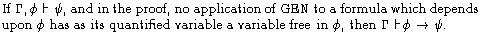In versions of quantificational logic utilizing a primive rule GEN (such as the present version), the Deduction Theorem requires a constraint. The condition in the statement of the Deduction Theorem, that no application of GEN to a formula which depends upon phi has as its quantified variable a variable free in phi, is designed to avoid fallacies that can arise from the application of GEN. For example, by a simple application of GEN, we know that there is a proof of the claim every x is such that exemplifies P from the claim x exemplifies P. However, we don't want to be able to then use the Deduction Theorem to prove if x exemplifies P, then every x is such that x exemplifies P. This, as we have seen, will be false whenever some but not all the members of the domain of quantification exemplify P (just let x be one of the things exemplifying P). We avoid such invalid applications of Conditional Proof by placing a constraint upon it. The constraint is stated in terms of the notion of dependence, which is defined in Principia Metaphysica in Logic/Dependence. Basically, the definition tells us that, in any proof, not only does a given formula appearing in the proof depend on itself, but moreover, so does any formula derived from the given formula by an application of Modus Ponens or Generalization. So in the proof of every x is such that x exemplifies P from x exemplifies P, the former depends on the latter. Thus, the Deduction Theorem does not apply, and we may not validly conditionalize the former on the latter.

Derived Rules: Universal Instantiation (UI)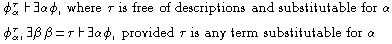Let us prove the first claim, namely, that any term free of descriptions and appropriately substitutable may be instantiated into a universal claim. Consider the following sequence of formulas: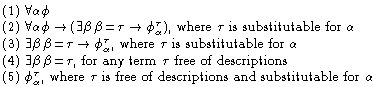This constitutes a proof of the first claim: (a) it is a finite sequence, (b) line 1 is the stated premise, line 2 is the first axiom of quantificational logic, (c) line 3 results from lines 1 and 2 by MP, (d) line 4 is the second axiom of quantificational logic, (e) line 5 results from lines 3 and 4 by MP. Notice also that by the Deduction Theorem, we can derive as a logical theorem the first axiom of classical quantification theory:Derived Rule: Universal Generalization (UG)This is the classic principle by which we may derive a universal generalization by reasoning with respect to an arbitrarily chosen member of the domain, using only facts generally true of all the members of the domain, thus taking care not to import any special fact that is unique to the arbitrarily chosen member into the proof. Under such constraints, if we can show that the arbitrarily selected object has a property, then it follows that every member of the domain as that property. We shall not prove this rule but rather show it in action. Let us prove the following using UG:Consider the following sequence of formulas: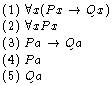Notice that this constitutes a proof of a exemplifies Q from everything that exemplifies P exemplifies Q and everything exemplifies P because: (a) it is a finite sequence, (b) lines 1 and 2 are members of the premise set, (c) line 3 results from line 1 by Universal Instantiation, (d) line 4 results from line 2 also by UI, and (e) line 5 results from lines 3 and 4 by MP. Notice also that in this proof, the constant symbol a did not occur in the premises and that the variable x does not occur in a exemplifies Q. So the UG applies and we are entitled to conclude that everything exemplifies Q is derivable from the two given premises.

Exercises: Look again at the derived rules of Existential Generalization and Instantiation. What invalid arguments are the restrictions on the rules designed to prevent? Find an introductory logic text and look at some examples of the rules in action. Then use them to demonstrate the following:## Some Further Consequences:

Logical Theorem:This first example simply asserts that if everything exemplifies P, then x exemplifies P is a theorem of logic. Exercise: Demonstrate that this is true.

Logical Theorem:If we assume the rule of Contraposition from sentential logic, then we may demonstrate this fact as follows. Consider first the following sequence of formulas:This constitutes a proof of if it is not the case that a exemplifies Q then it is not the case that a exemplifies P from the universal claim everything is such that if it exemplifies P then it exemplifies Q. In this proof, line 3 follows from line 2 by the Rule of Contraposition. Given this proof, notice that the conditions for applying the rule of Universal Generalization are met. So by UG, we know that there is a proof of everything is such that if it doesn't exemplify Q it doesn't exemplify P from everything is such that if it exemplifies P then it exemplifies Q. But by the Deduction Theorem, it follows that if we conditionalize the former on the latter, the result is a theorem of logic.